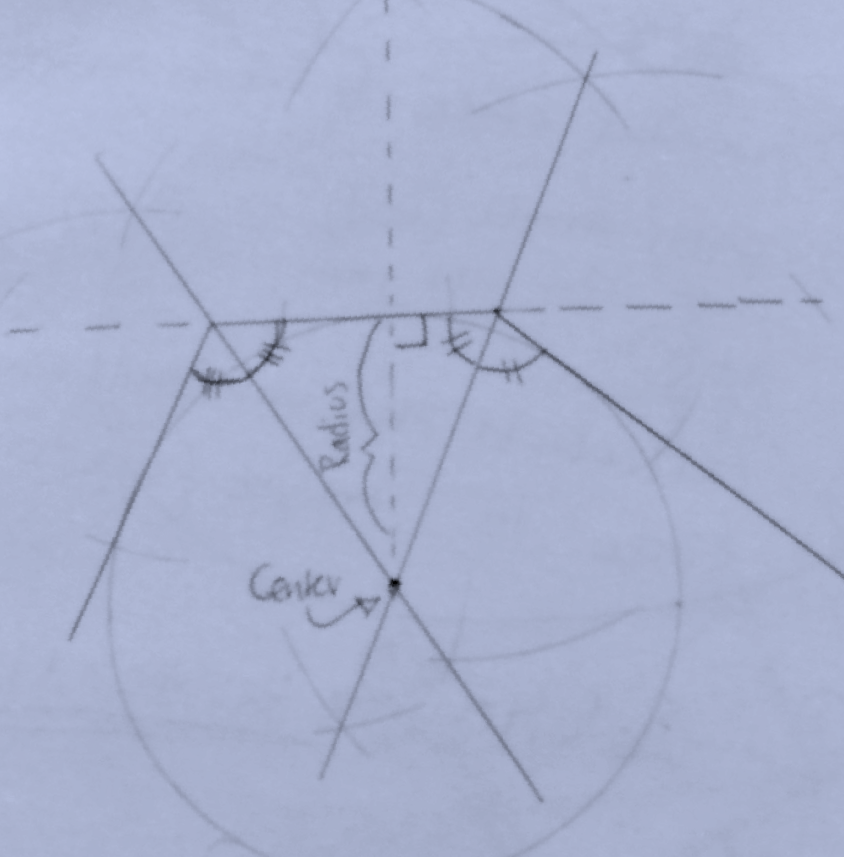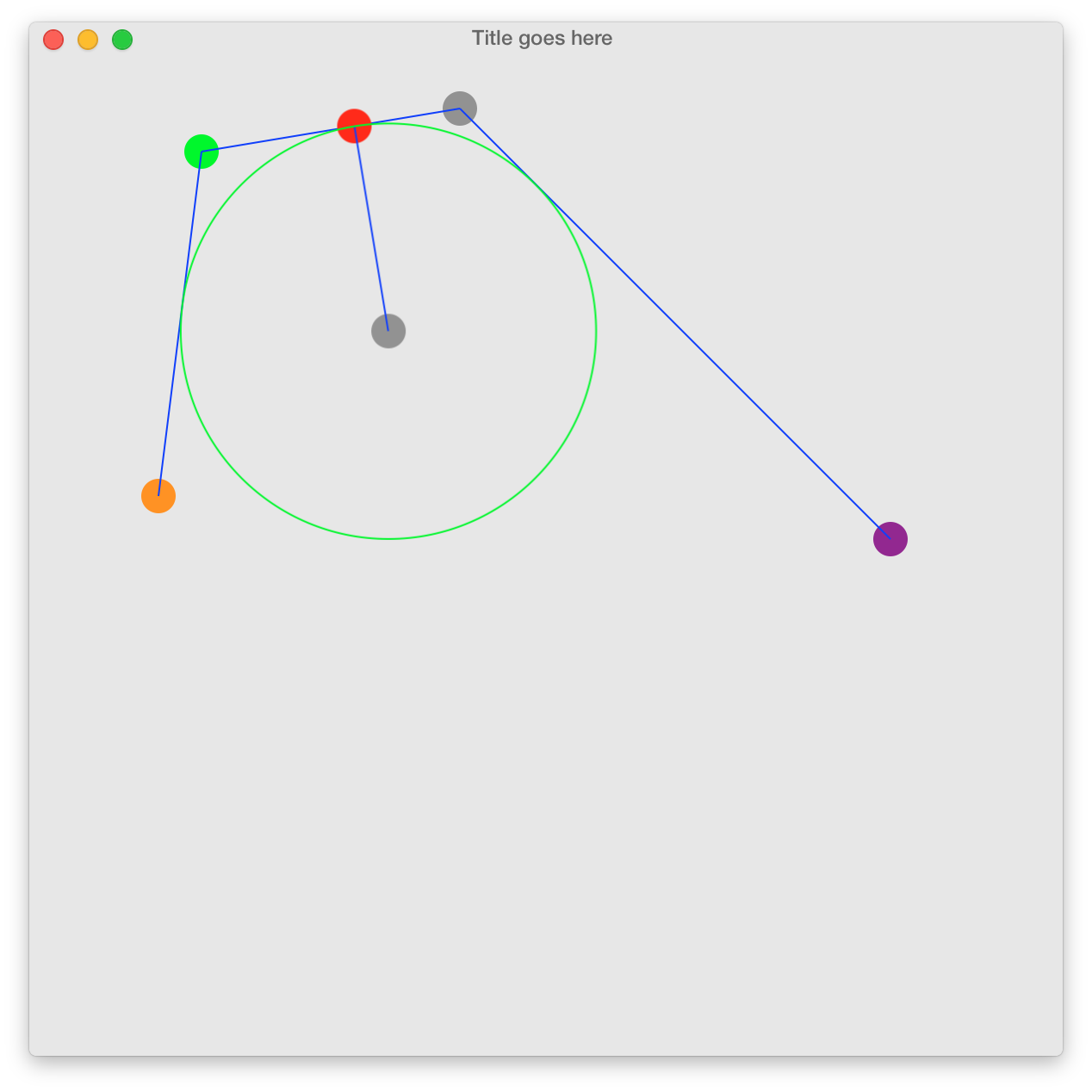Fitting a circle Given 4 points. p1,p2,p3,p4

#### The steps:

1. The converging point of the bisector angles for p2 and p3 gives you the centre of the circle.
2. To find the radius: Project the orthogonal normal from the centre onto the line (p2,p3)
3. Draw the circle with the centre and the radius

(You can use a compass and a ruler to test this theory out)#### The result came out like this in swift code:The above solution can be discussed here: https://math.stackexchange.com/questions/656497/find-a-circles-radius-with-three-known-tangent-lines/1907482#1907482